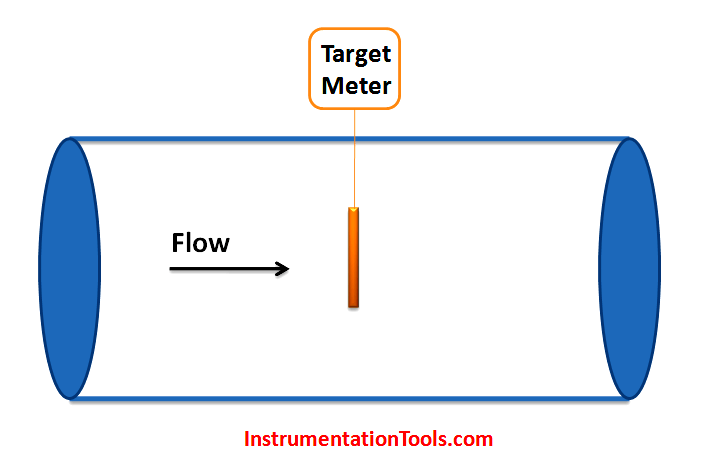# Target Flow Meter Working Principle

Target flow meters, also known as drag force flow meters, insert a target (drag element), usually a flat disc or a sphere with an extension rod, into the flow field. They then measure the drag force on the inserted target and convert it to the flow velocity.

## Target Flow MetersTarget flow meters measure flow by measuring the amount of force exerted by the flowing fluid on a target suspended in the flow stream. The force exerted on the target by the flow is proportional to the pressure drop across the target.

Similar to differential pressure flow meters, Bernoulli’s equation states that the pressure drop across the target (and hence the force exerted on the target) is proportional to the square of the flow rate.

One major advantage of the target flow meter over other flow meters is, with a sphere drag element, a proper strain gage layout, and well thought-out mathematical formulas, a target flow meter is capable of measuring sporadic and multi-directional flows.The deflection of the target and the force bar is measured in the instrument.

The force on the target can be expressed as :

#### F = cd ρ v2 At / 2

where

F = force on the target (N)

cd = overall drag coefficient obtained from empirical data

ρ = density of fluid (kg/m3)

v = fluid velocity (m/s)

At = target area (m2)

• Low initial set up cost
• Can be used with a wide variety of fluids, even viscous fluids and slurries.
• Can be used in abrasive, contaminated, or corrosive fluid flow
• Can be made to measure flow velocity that is sporadic or multi-directional with sphere drag element designs

• Pressure drop is inevitable due to the rod and the drag element
• Less popular than it was before

#### Articles You May Like :

Vortex Flow Meter Sensors

Optical flow meter Principle

Why Turndown Ratio is important ?

Non-intrusive Flow Instruments

Ultrasonic Flow Meter Principle

Don't Miss Our Updates
Be the first to get exclusive content straight to your email.
We promise not to spam you. You can unsubscribe at any time.

### 4 thoughts on “Target Flow Meter Working Principle”

1.Thanks ..

2.Thanks for this…

3.4.